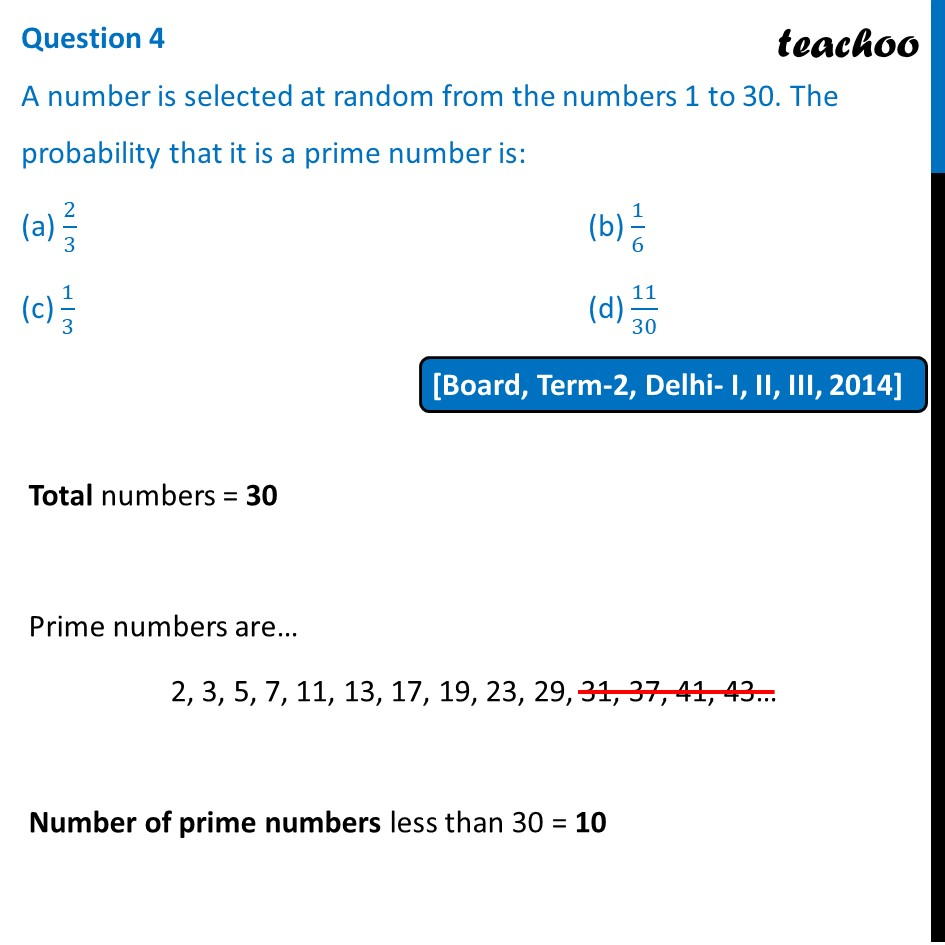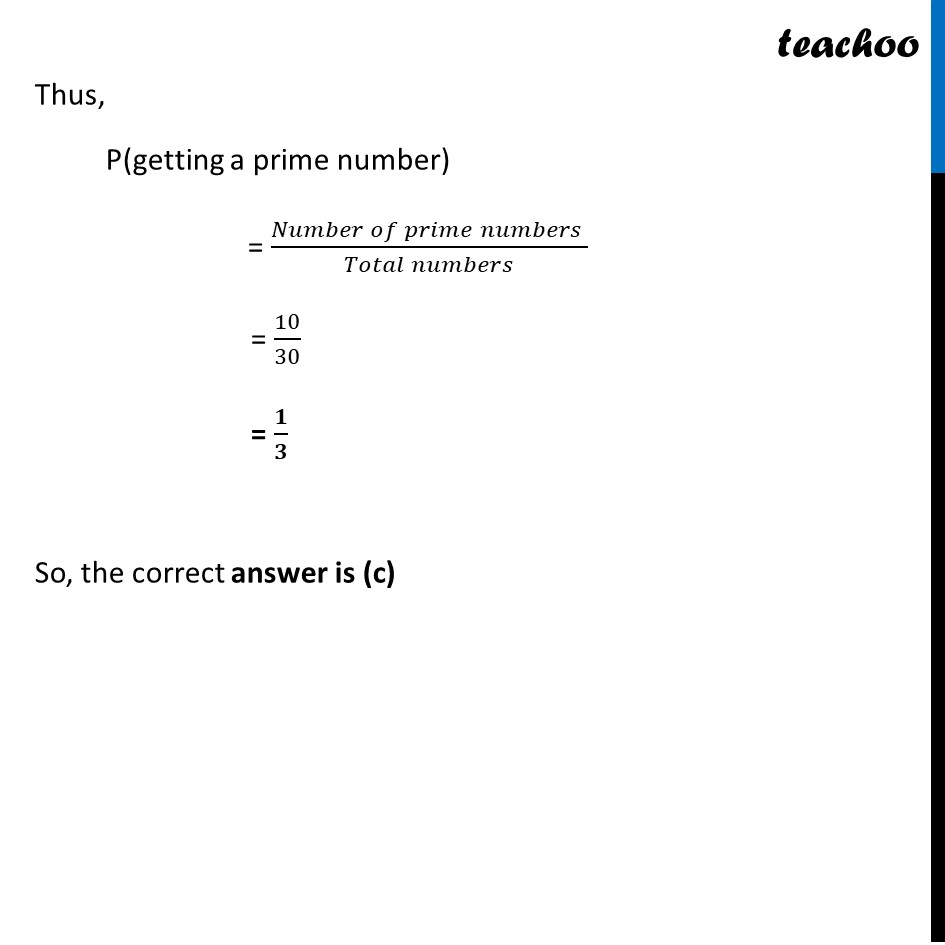Past Year MCQ

Chapter 14 Class 10 Probability
Serial order wiseLearn in your speed, with individual attention - Teachoo Maths 1-on-1 Class

### Transcript

Question 4 A number is selected at random from the numbers 1 to 30. The probability that it is a prime number is: (a) 2/3 (b) 1/6 (c) 1/3 (d) 11/30 Total numbers = 30 Prime numbers are… 2, 3, 5, 7, 11, 13, 17, 19, 23, 29, 31, 37, 41, 43… Number of prime numbers less than 30 = 10 Thus, P(getting a prime number) = (𝑁𝑢𝑚𝑏𝑒𝑟 𝑜𝑓 𝑝𝑟𝑖𝑚𝑒 𝑛𝑢𝑚𝑏𝑒𝑟𝑠 )/(𝑇𝑜𝑡𝑎𝑙 𝑛𝑢𝑚𝑏𝑒𝑟𝑠) = 10/30 = 𝟏/𝟑 So, the correct answer is (c)